# 1.1 Introduction

• Regression analysis is a statistical tool used to explain the relationship between a response (dependent, outcome) variable as a function of one or more predictor (independent) variables.

Here are some examples of relationships between independent variables and dependent variables:

1. Employee efficiency may be related to years of training, educational background, and age of the employee.
1. The amount of time until a headache clears, when taking a pain killer, may be related to the dosage, age, and gender.
1. The number of votes for a presidential candidate for each city may be related to the candidate’s gender, city average income, and the state.
1. In a study of schools in California, student academic performance for each school may be related to the poverty level of students in the school, the number of student enrolled in the school, the percentage of teachers with full credentials in the school, or the number of students in the class.
• In a linear regression model we assume this relationship can be explained with a linear function.
• a simple regression model has one response and a single predictor.

# 1.2 Linear function

• The data in a simple regression model are observed in pairs. For example:

$$X$$: The predictor. $$x_1, x_2, \dots, x_n$$ are $$n$$ observations from $$X$$.

$$Y$$: The response. $$y_1, y_2, \dots, y_n$$ are $$n$$ observations from $$Y$$.

each index represent one case or unit of observation in the data.

If we plot these points against the X and Y axis, our goal is to fit the best line to the data.

A linear function can be determined by the intercept and the slope of the line.

The figure below shows a plot of linear function

$y = f(x) = 1 + 0.5 \times x$

The intercept is equal to 1 and slope of the line is equal to 0.5.

• The intercept represents the value of y given x = 0.
• The slope represents the increase in y for a one unit increase in the x.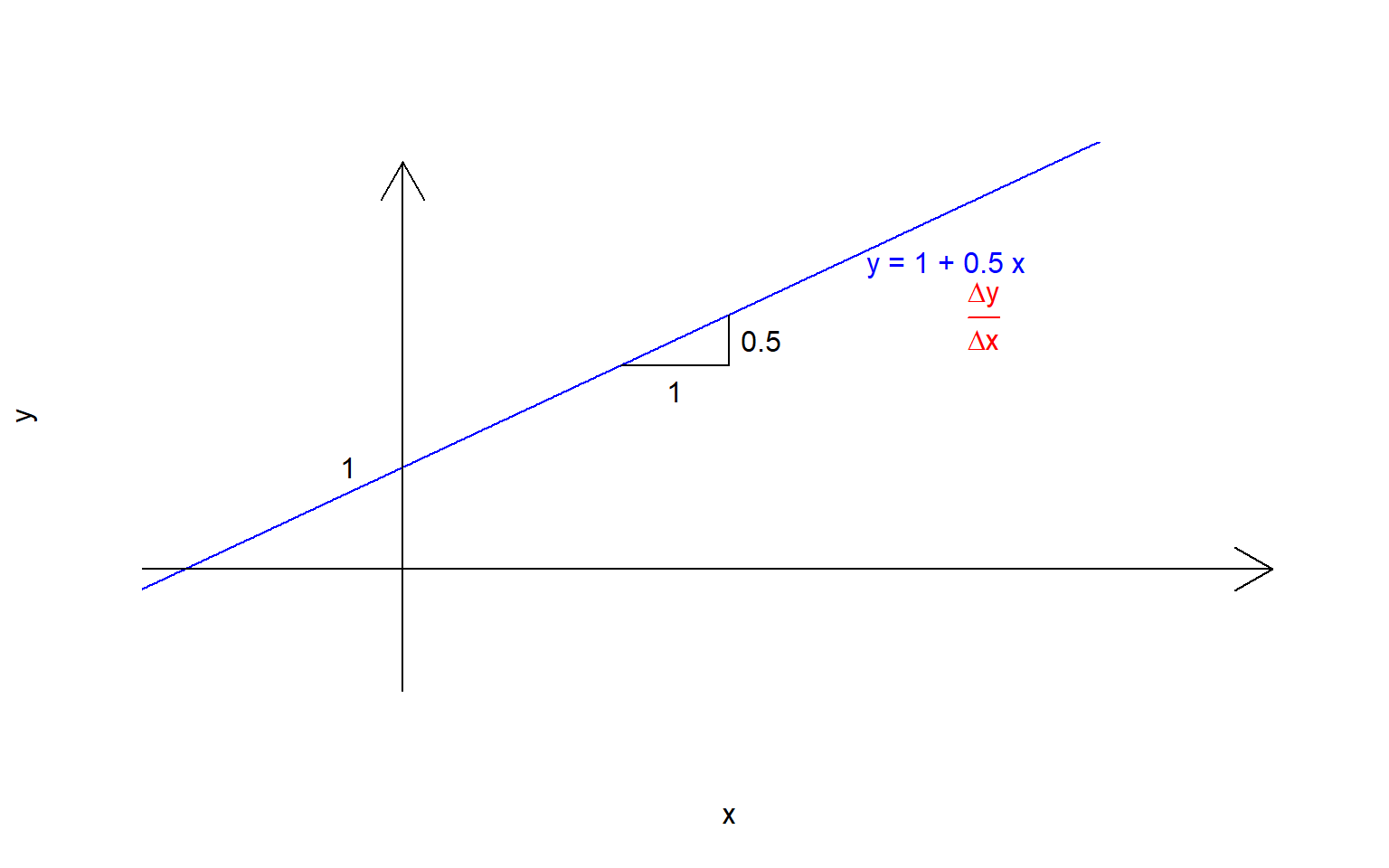# 1.3 Linear regression as a statistical model

In real life situations, we usually cannot explain $$Y$$ as an exact function of $$X$$. There is always some error or noise in the dependent variable that cannot be explained perfectly by the relationship between the independent and dependent variables. We call this the error term in the model. We represent the formula for a simple linear model as:

$Y = \beta_0 + \beta_1 X + \epsilon$

Where $$\beta_0$$ is the intercept, $$\beta_1$$ is the slope of the model, and $$\epsilon$$ is the error term.

• The regression model has two parts: a linear function and an error term.

• The quantities $$\beta_0$$ and $$\beta_1$$ are called model parameters and they are estimated from the data.

• The linear function predicts the mean value of the outcome for a given value of the predictor variable.
• The mean value or expected value of a variable $$Y$$ is noted as $$\text{E}(Y)$$ and the mean of $$Y$$ conditional on $$X = x$$ is written as $$\text{E}(Y | X = x)$$ and reads “the expected value of $$Y$$ given $$X = x$$”.
• In a simple linear regression, the mean function of the outcome is given by

$E(Y |X = x) = \beta_0 +\beta_1x$

• The error term is the unexplained uncertainty of the model and is a random variable with mean equal to zero and unknown variance $$\sigma ^2$$.
• The error term usually, but not necessarily, is assumed to have a normal distribution.
• In a simple regression model, for each individual or unit $$i = 1, 2, \cdots, n$$ in the study, we have a pair of observations, $$(x_i, y_i)$$.

For example, if we want to study the relationship between api00, a school-wide measure of academic performance, and the number of students enrolled in a school, the data might look like this:

index api00 enroll
1 693 247
2 570 463
3 395 546
. . .
. . .
. . .
n 478 520

# 1.4 Ordinary least squares

• If we plot the pairs in a two dimensional x-y axis it is called a scatter plot.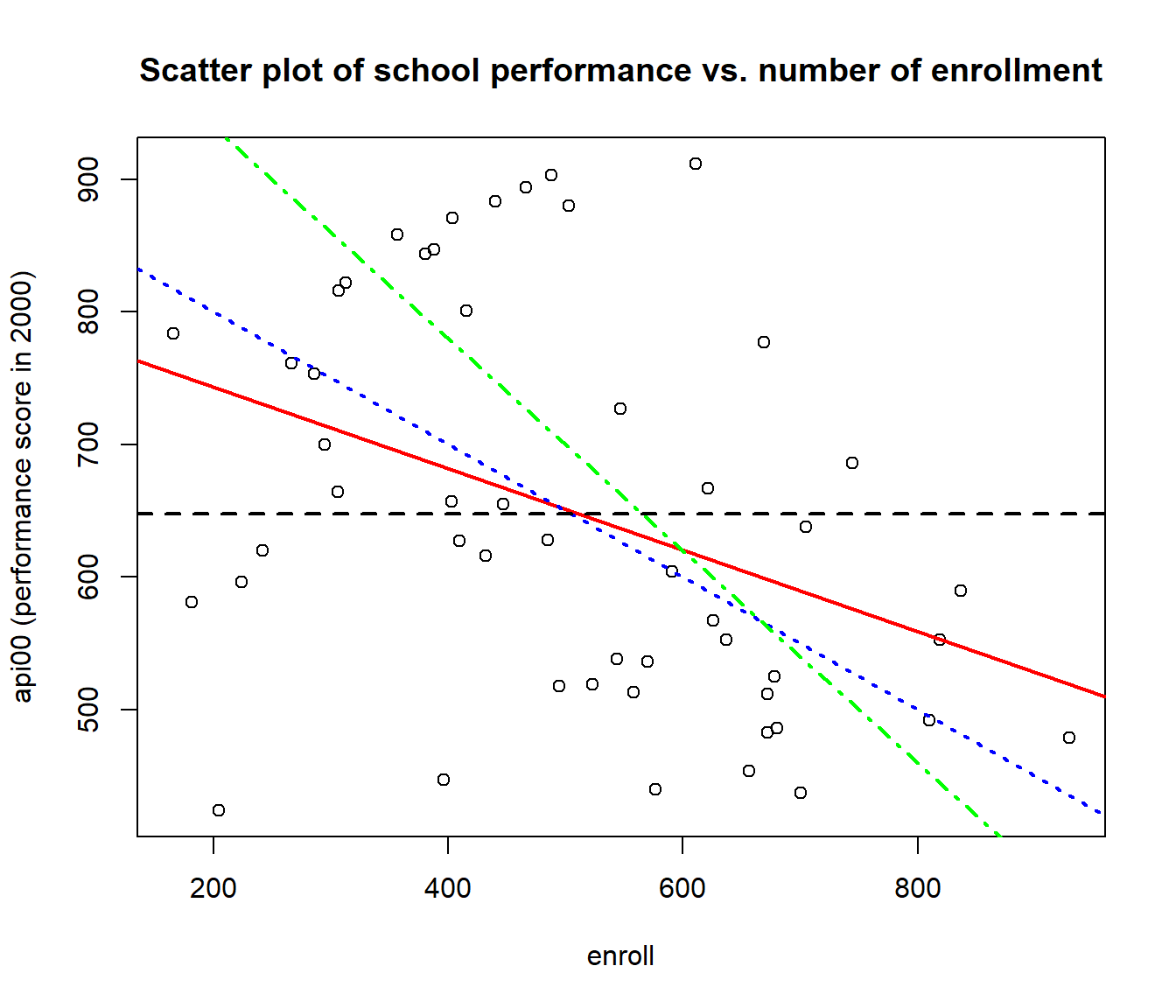• Each of the lines in the graph above could be a fit to the data.
• The goal is to estimate parameters $$\beta_0$$ and $$\beta_1$$, the intercept and the slope of the line, such that the line fits the best with the data.

• In order to have unbiased estimation for model parameters, the mean of the error term must be equal to zero.

• A method to achieve this best fitted linear function is called Ordinary Least Square (OLS) method.

• The assumptions of the OLS method are:

1 - Errors have mean equal to zero.

2 - Errors are independent.

3 - Errors have equal variances (Homoscedasticity).

4 - Errors follow normal distribution.

5 - The relationship of dependent variable with the independent variables is linear.

• Estimate model parameters are noted as $$\hat{\beta}_0$$ and $$\hat{\beta}_1$$.

• The estimated model will be

$\hat{y} = \hat{\beta}_0 + \hat{\beta}_1 x$

• $$\hat{y}$$ represents the predicted mean of the outcome for a given value of $$x$$. We also call it the fitted value.
• The predicted value for each observation can be calculated by the estimated line as: $$\hat{y_i} = \hat{\beta}_0 + \hat{\beta}_1 x_i$$
• The difference between the observed value of $$y_i$$ and the predicted value $$\hat{y_i}$$ is called the residual which is:

$e_i = y_i - \hat{y_i}$

• In OLS we estimate the parameters of the model (intercept and slope) by minimizing the sum of squares of the residuals.

$\min \sum_{i = 1}^{n} (y_i - \hat{y_i}) ^ 2$

*Note:

• The predictor variable may be continuous, for example, age or height. Or it may be a categorical variable with two or more levels (entered as dummy variables).
• The response or dependent variable in OLS is assumed to be continuous.
• There are situations where we have a discrete variable as outcome, for example count data. In these cases we need to be cautious when we use OLS.
+ Two main issues when the outcome is not continuous is that we may violate homogeneity of variance and also the predicted values might be out of the range of possible values for the outcome (e.g. negative values for a count outcome).
• In regression, we should generally use the model to predict outcomes at values within the observed range of the predictor variables.

• For example if we are modeling blood pressure using BMI as predictor, we should use our model to predict blood pressure for people with BMI within the range of observed values of BMI in the data that we used to estimate the model.

# 1.5 Decomposing the sum of squares and ANOVA

Regression can be seen as a model to explain variability of the response variable using the predictor variable(s). We will show how we can decompose the total variation in the response into explained and unexplained variation.

Total Variation

If only the intercept is used to model the response variable (slope restricted to be zero), the regression line will be a horizontal line at the mean of the response variable.

In our example this will be the mean of api00 (performance score in 2000), which is the line $$y = \bar{y} = 649.7647$$

From this intercept model, the residuals for each data point $$i$$ will be $$y_i - \bar{y}$$.

The sum of the these residuals squared, $$\sum_{i = 1}^{n} (y_i - \bar{y})^2$$, is the Total Sums of Squares (TSS), representing the total variation in the outcome.

Decomposing into Unexplained and Explained Variation

When we add a predictor to the model, we can decompose the total variation into variation explained by the predictor and unexplained variation.

We can decompose the residual from the intercept model, $$y_i - \bar{y}$$, into two parts using the fitted value, $$\hat{y_i}$$, from the regression of api00 by predictor, enroll.

This can be written as:

$y_i - \bar{y} = (y_i - \hat{y_i}) + (\hat{y_i} - \bar{y})$

Where $$\bar{y}$$ is the mean of response, $$\hat{y_i}$$ is the fitted value from the regression model that includes the predictor variable enroll and $$y_i$$ is the observed response.

For example, if school $$i$$ has enrollment=$$810$$, then we can decompose the deviation of the api score for this school from the mean api score (the residual):

$\text{api00}_i - \text{mean(api00)} = (\text{api00}_i - \hat{\text{api00}_i}) + (\hat{\text{api00}_i} -\text{mean(api00)})$

The graph below shows the decomposition of the residual into two parts.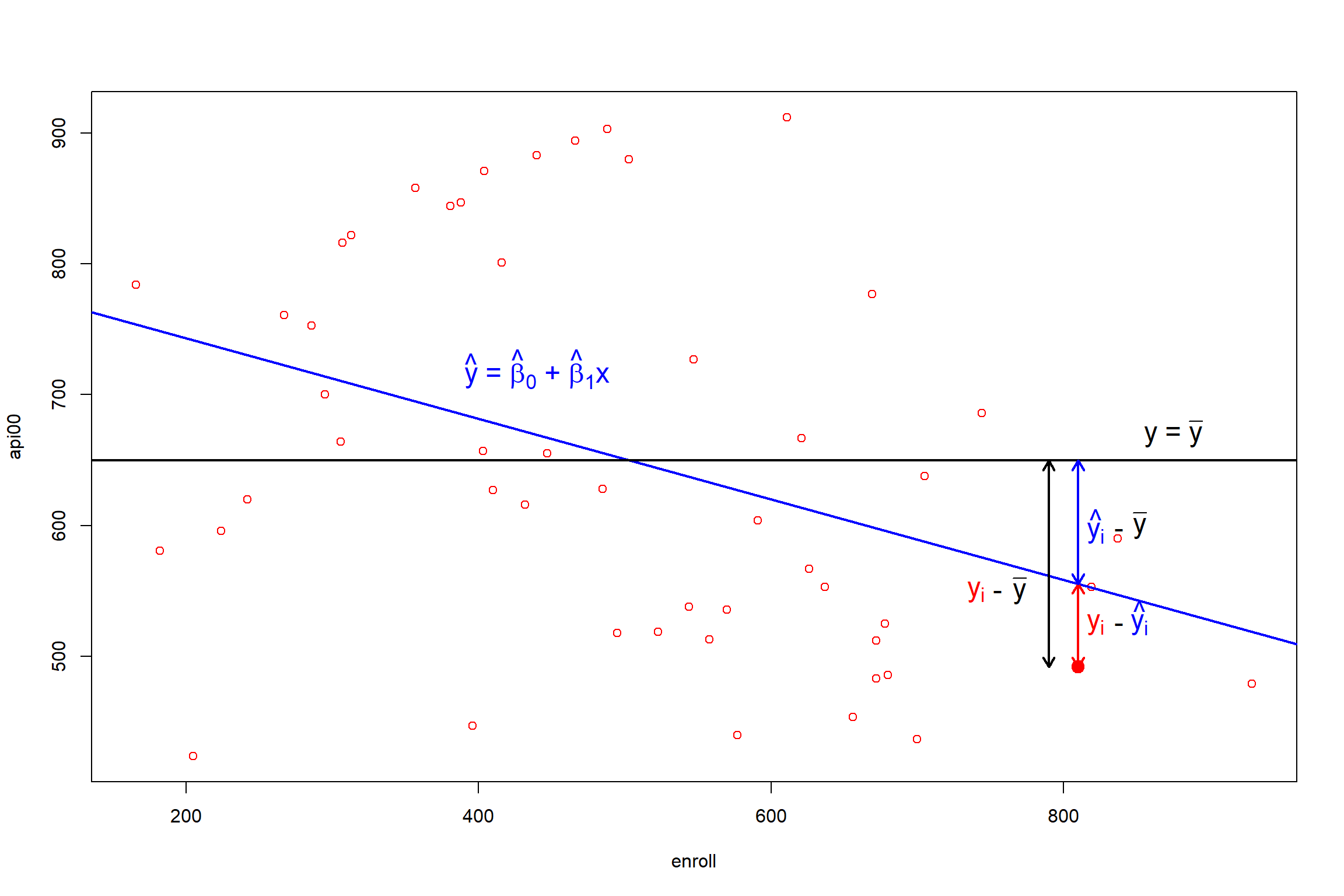It can be shown the following equation is true:

$\sum_{i = 1}^{n} (y_i - \bar{y})^2 = \sum_{i = 1}^{n}(y_i - \hat{y_i})^2 + \sum_{i = 1}^{n}(\hat{y_i} - \bar{y})^2$ $\text{Total variation of the outcome} = \text{unexplained variation} + \text{variation explained by the model}$

The left hand side of this equation is called Total Sum of Square (TSS). The first term of the right hand side of the above equation is called Residual Sum of Square (RSS) and the second term is called Sum of Square Regression (SSreg). Thus, we have:

$TSS = RSS + SSreg$

The ratio of SSreg to SST is called $$R^2$$. $$R^2$$ is the proportion of the total variation that can be explained by the model.

$R^2 = \frac{SSreg}{TSS} = 1 - \frac{RSS}{TSS}$

*In some texts $$RSS$$ is also noted as $$SSE$$ or sum of squares of errors.

• You might also have seen this decomposition of the sums of squares in analysis of variance (ANOVA).

• $$R^2$$ is between 0 and 1. Higher $$R^2$$ means the model explains more of the variation of the outcome.

# 2.1 A quick overview of RStudio environment

Before we begin, let’s take a look at the RStudio environment. RStudio is an integrated development environment (IDE) designed to make R easier to use. The RStudio interface has four panes: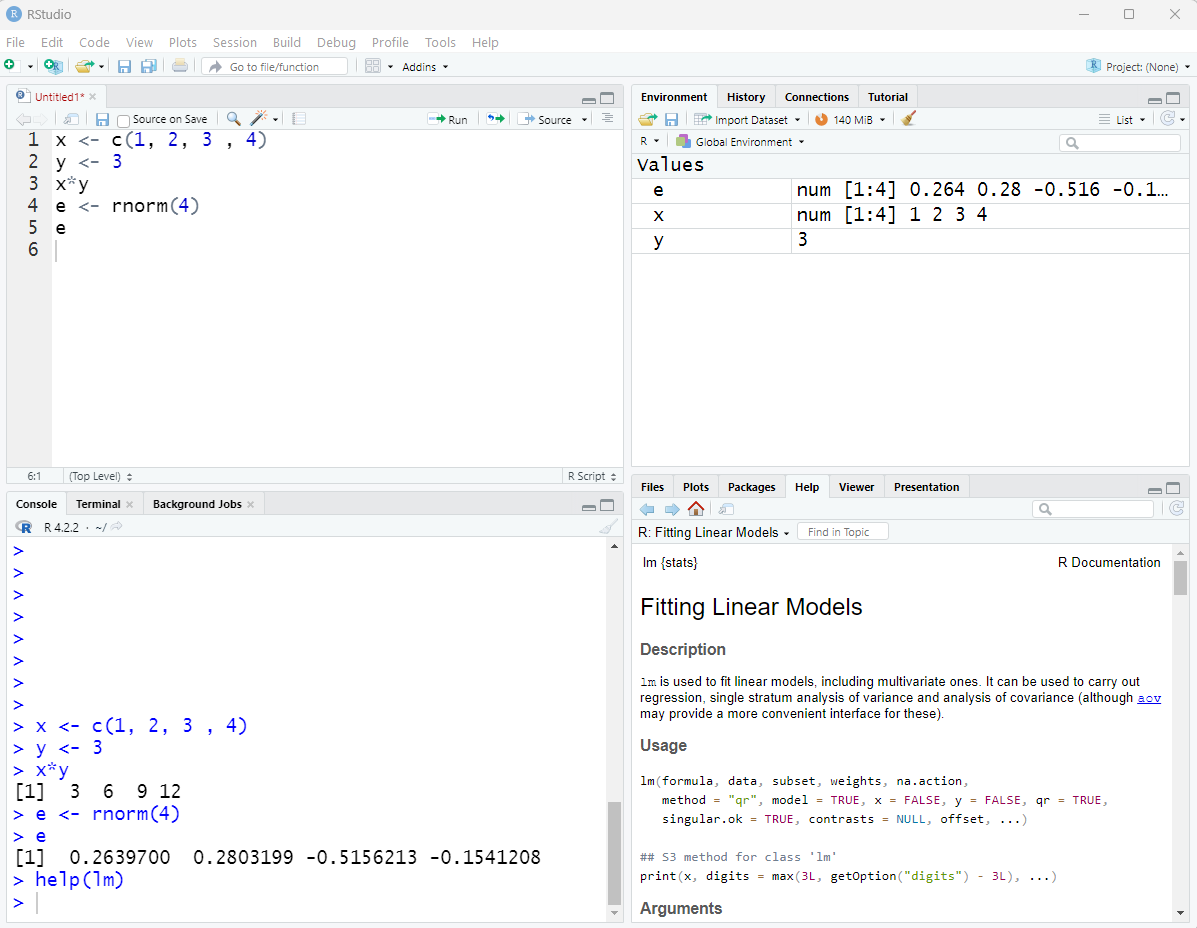RStudio Panes

• Source code (R Script) editor: to type, edit and manage R commands. The first time you open RStudio the source code pane is hidden and will appear after we open a new or existed R script. R scripts are text files with the .R extension. You can save R script file to keep R code that you have written.

• To run a command or a set of commands from the R Script, select them first and hold Ctrl + Enter Enter.
• Console: when we run a command from an R Script, its output adn results (and error and warning messages) appear in the console. We can also type a command in the console and click Enter to run it directly from the R console.

• Environment and History: One pane usually includes tabs for Environment and History. The Environment tab shows R objects we define in the current R session, for example, vectors, matrices, and data frames. The History tab simply shows all commands that we already run and evaluated in the R console.

• Files, Plots, Packages, and Help: The final pane includes multiple useful tabs. We can always customize R panes and include tabs that we need.

• Files tab is used to view and manage directories and files in the hard drive of the computer. From the Files tab we can navigate to a directory and set it as the working directory.

• Plots tab is used to view and manage plots. By clicking on the export we can save our plots, for example as jpeg or PDF.

• Packages shows a list of all the R packages installed on the computer. We can load a package by checking their box. Also we can install a new package from this tab.

• However, another way to manage R packages is to run function install.packages("package_name") in the R console or from an R script to install a new package and function library(package_name) to load a package.
• Help tab displays the help documentation for an R function. We can use search to search help from this tab or we can use ?function_name or help (function_name) from the console.

Now let’s read our data set into the R workspace. The data set used in this portion of the seminar is downloadable from the link below:

elemapi2v2

You can download the data and save it into your local computer and load it from there, or you can directly read it from the web URL address. The data is in .csv format, and we use the read.csv function to read and load it in R. The resulting output will be a data.frame object that will appear in the environment tab. By clicking on the data.frame object in the environment pane we can view it as a spreadsheet.

The following code is use to read and load the data into the R workspace first from the URL address and then from the local computer:

#reading the data file in  R
#We can also read the data from the local computer
#we can set the working directory by setwd()
#                header = TRUE, sep = ",")

An object’s type can be determined by the function class. To get the names of variables in a data.frame we use names and to get number of rows and columns of data.frame we use dim.

#class of object d, returns data.frame
class(d)  
##  "data.frame"
#returns names of variables (columns)
names(d) 
##   "snum"     "dnum"     "api00"    "api99"    "growth"   "meals"
##   "ell"      "yr_rnd"   "mobility" "acs_k3"   "acs_46"   "not_hsg"
##  "emer"     "enroll"   "mealcat"  "collcat"  "abv_hsg"  "lgenroll"
 #returns number of rows and columns of data.frame
dim(d)   
##  400  24

As we mentioned the output of read.csv is a data.frame object. A data.frame is a list of variables with the same number of rows with unique row names. In the data.frame d we have 24 variables and 400 observations.

# 2.3 Exploring the data

As researchers we need to make sure first that the data we cleaned hold plausible values. Descriptive analysis will help us to understand our data better and investigate if there are any problems in the data. In this workshop we skip this part, but in a real study descriptive analysis is an important part of a good data analysis.

Here we take a look at structure of the data and summary statistics. We only show the first 4 variables.

#returns structure of variables in the data frame d
str(d)
#Summary statistics of variables in d
summary(d)  
## 'data.frame':    400 obs. of  4 variables:
##  $snum : int 906 889 887 876 888 4284 4271 2910 2899 2887 ... ##$ dnum : int  41 41 41 41 41 98 98 108 108 108 ...
##  $api00: int 693 570 546 571 478 858 918 831 860 737 ... ##$ api99: int  600 501 472 487 425 844 864 791 838 703 ...
##       snum           dnum           api00           api99
##  Min.   :  58   Min.   : 41.0   Min.   :369.0   Min.   :333.0
##  1st Qu.:1720   1st Qu.:395.0   1st Qu.:523.8   1st Qu.:484.8
##  Median :3008   Median :401.0   Median :643.0   Median :602.0
##  Mean   :2867   Mean   :457.7   Mean   :647.6   Mean   :610.2
##  3rd Qu.:4198   3rd Qu.:630.0   3rd Qu.:762.2   3rd Qu.:731.2
##  Max.   :6072   Max.   :796.0   Max.   :940.0   Max.   :917.0
• Names and descriptions of the data set variables are in the following table.
Variable name Description
snum school number
dnum district number
api00 api 2000, Academic performance index in 2000
api99 api 1999, Academic performance index in 1999
growth growth from 1999 to 2000
meals percentage of students who gets free meals
ell english language learners
yr_rnd year round school
mobility percentage 1st year in school
acs_k3 avg class size k-3
acs_k3 avg class size k-3
acs_46 avg class size 4-6
not_hsg parent not hsg
hsg parent hsg
some_col parent some college
avg_ed avg parent ed
full percentage teachers with full credential
emer percentage of teachers with emergency credential
enroll number of students
mealcat Percentage free meals in 3 categories
. .
. .
. .

# 2.4 lm function

• In R we use function lm to run a linear regression model. Let’s look at R help documentation for function lm
help(lm)   #shows R Documentation for function lm()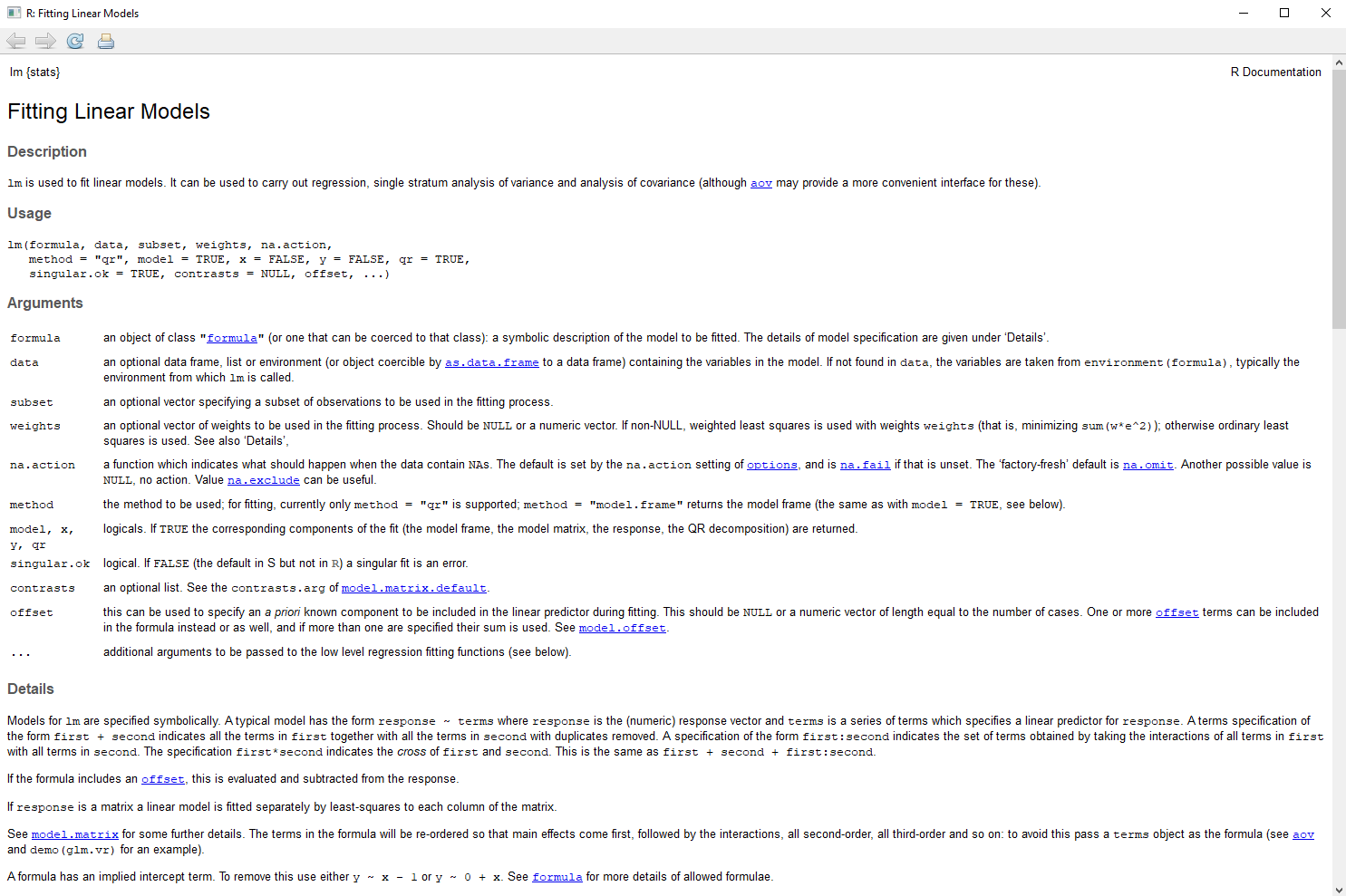# 2.5 Our first linear model in R

Now that we have reviewed the linear model, let’s use R and run a simple regression model.

• In our data example we are interested to study the relationship between student academic performance and characteristics of the school. For example we can use variable api00, a school-wide measure of academic performance, as the outcome, and variable enroll, the number of students in the school, as the predictor.

• We expect that better academic performance would be associated with lower numbers of students enrolled in the school.

#Simple regression model of DV api00 and IV enroll
m1 <- lm(api00 ~ enroll, data = d)
class(m1)
##  "lm"
print(m1)    #Prints coefficients of the model
##
## Call:
## lm(formula = api00 ~ enroll, data = d)
##
## Coefficients:
## (Intercept)       enroll
##    744.2514      -0.1999
• The first argument to the function lm() is the formula for the model. The response variable is on the left side of "~" and the predictor variable is on the right side.
+    api00 ~ enroll is interpreted as outcome api00 is modeled by the predictor enroll.
• data = d tells the lm function that the variables in the formula are in the data.frame d.
• The result of function lm() will be passed to m1 as a lm object.

• print() prints the estimated coefficients of the model.

• The estimated linear function is:

$\hat{\text{api00}} = \text{744.2514 - 0.1999 enroll}$

• The coefficient for enroll is $$-0.1999$$, or approximately $$-0.2$$, meaning that for a one unit increase in enroll, we would expect a $$0.2$$ unit decrease in api00. For example, a school with 600 students would be expected (on average) to have an api00 score 20 units less than a school with 500 students.

• The intercept is $$744.2514$$, and this is the predicted mean api00 value when enroll equals zero. In most cases, the constant is not very interesting.

# 2.6 Summary of the lm object

To observe the result of the lm object in more detail, we use the summary() function:

#Prints summary of the model
summary(m1)    
##
## Call:
## lm(formula = api00 ~ enroll, data = d)
##
## Residuals:
##     Min      1Q  Median      3Q     Max
## -285.50 -112.55   -6.70   95.06  389.15
##
## Coefficients:
##              Estimate Std. Error t value Pr(>|t|)
## (Intercept) 744.25141   15.93308  46.711  < 2e-16 ***
## enroll       -0.19987    0.02985  -6.695 7.34e-11 ***
## ---
## Signif. codes:  0 '***' 0.001 '**' 0.01 '*' 0.05 '.' 0.1 ' ' 1
##
## Residual standard error: 135 on 398 degrees of freedom
## Multiple R-squared:  0.1012, Adjusted R-squared:  0.09898
## F-statistic: 44.83 on 1 and 398 DF,  p-value: 7.339e-11
• The first part Call: shows the model specification.

• The second part Residuals: displays the five number summary statistics of the model residuals.

• In the Coefficients: section, we have a table of estimated coefficients, standard errors, t-values, and p-values for the hypothesis test that a coefficient equals zero.

• Stars (and .) are used as short-hand indicators for p-values according to the table below:
p-value symbols
p < 0.001 ***
0.001 < p < 0.01 **
0.01 < p < 0.05 *
0.05 < p < 0.1 .
p > 0.1
• Here both the intercept and slope are statistically significant.
• In the last portion of the output we see:

• Residual standard error is 135 and its degrees of freedom equal to 398.
• Multiple R-squared is the $$R^2$$ of the model, equal to 0.1012, and adjusted R-squared is 0.09898 which is adjusted for number of predictors.
• In the simple linear regression model $$R^2$$ is equal to the square of the correlation between the response and predictor variables. We can run the function cor() to confirm this.
#correlation coefficient of api00 and enroll
r <- cor(d$api00, d$enroll)
#this is equal to r-squared in simple regression
r ^ 2                      
##  0.1012335
• The last line gives the overall F-statistic, testing the fit of the current model against the null model, the model with only an intercept. $$F=44.83$$ with 1 and 398 degrees of freedom and with p-value equal to 7.339e-11. This p-value in a simple regression model is exactly equal to p-value of the slope.

# 2.7 Components of lm-class objects and post-estimation for regression models in R

• As we said the output of the lm() function is an object of class lm. We can use functions ls() or str() to list the components of object m1.
ls(m1)        #list of components in object class lm
##   "assign"        "call"          "coefficients"  "df.residual"
##   "effects"       "fitted.values" "model"         "qr"
##   "rank"          "residuals"     "terms"         "xlevels"
• To access to the above components we can use $ sign after the lm object name. m1$coefficients        #returns coefficients of the model
## (Intercept)      enroll
## 744.2514142  -0.1998674
m1$fitted.values[1:10] #a vector of fitted values here we show the first 10 ## 1 2 3 4 5 6 7 8 ## 694.8842 651.7128 665.3038 660.7068 640.3203 675.6969 683.6916 441.8520 ## 9 10 ## 612.3389 671.8994 We can store the extracted components in a new object. #a vector of residuals residuals <- m1$resid  
• There are several extractor functions that can be applied to a lm-class object to extract one of its components. We use coefficients() or coef() to extract the model coefficients:
#r coeff function
coefficients(m1)
## (Intercept)      enroll
## 744.2514142  -0.1998674

To get the confidence intervals for the coefficients of the model, we use confint()

confint(m1) # returns a matrix of Confidence Interval for coefficients
##                   2.5 %      97.5 %
## (Intercept) 712.9279024 775.5749259
## enroll       -0.2585532  -0.1411817
• We can use function anova() to extract the sums of squares of the model.
anova(m1)  #anova table
## Analysis of Variance Table
##
## Response: api00
##            Df  Sum Sq Mean Sq F value    Pr(>F)
## enroll      1  817326  817326  44.829 7.339e-11 ***
## Residuals 398 7256346   18232
## ---
## Signif. codes:  0 '***' 0.001 '**' 0.01 '*' 0.05 '.' 0.1 ' ' 1

In ANOVA table we have the sum of squares of the regression model in the row named “enroll” and sum of squares of residuals with their degrees of freedom in the row below. The table also shows the F-statistic that we have seen before in the summary of the model. Mean squares are sum of squares divided by their degrees of freedom.

• Another important function is predict() which can be use to get predicted value for new data points.
#first we need to create new data.frame including all predictors of the model
new.data <- data.frame(enroll = c(500, 600, 700))
predict(m1, newdata = new.data)
##        1        2        3
## 644.3177 624.3309 604.3442
• Optionally we can use other R packages for example sjPlot to create a nice table for regression results. We are not going to discuss it in detail in this workshop.
#Optional if someone likes to use nice table for regression results
#install.packages(sjPlot)
library(sjPlot)
tab_model(m1)
api00
Predictors Estimates CI p
(Intercept) 744.25 712.93 – 775.57 <0.001
enroll -0.20 -0.26 – -0.14 <0.001
Observations 400
R2 / R2 adjusted 0.101 / 0.099

# 2.8 Plotting a scatter plot and the regression line

In addition to getting the regression table and statistics, it can be useful to see a scatter plot of the predicted and outcome variables with the regression line plotted.

plot(api00 ~ enroll, data = d) #scatter plot of api00 vs. enroll
abline(m1, col = "blue")       #add regression line to the scatter plot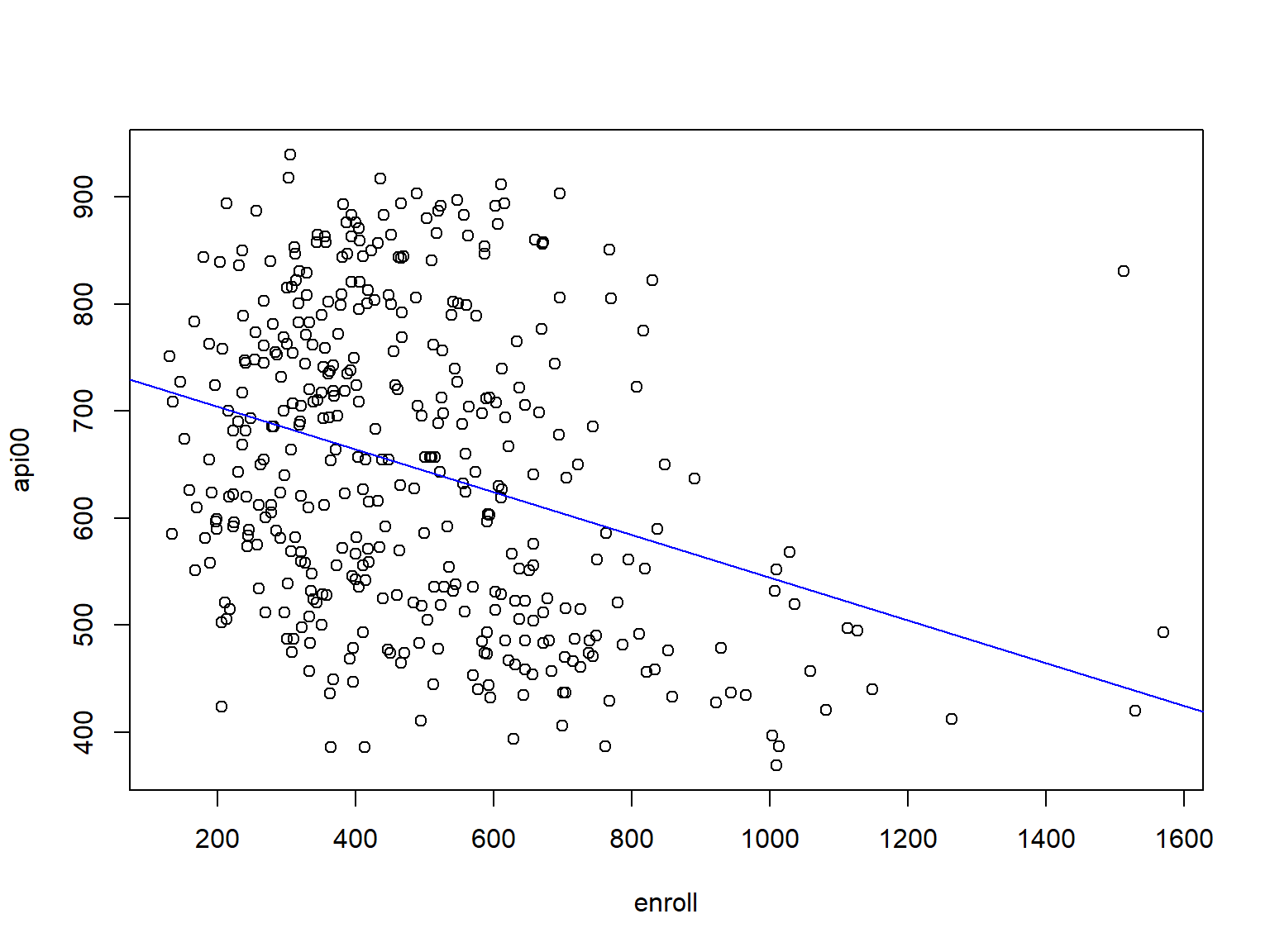As you see, some of the points appear to be outliers. If you add text with labels = d$snum on the scatter, you can see the school number for each point. This allows us to see, for example, that one of the outliers is school 2910. #adding labels(school number) to the scatter plot plot(api00 ~ enroll, data = d) text(d$enroll, d$api00+20, labels = d$snum, cex = .7)
abline(m1, col = "blue")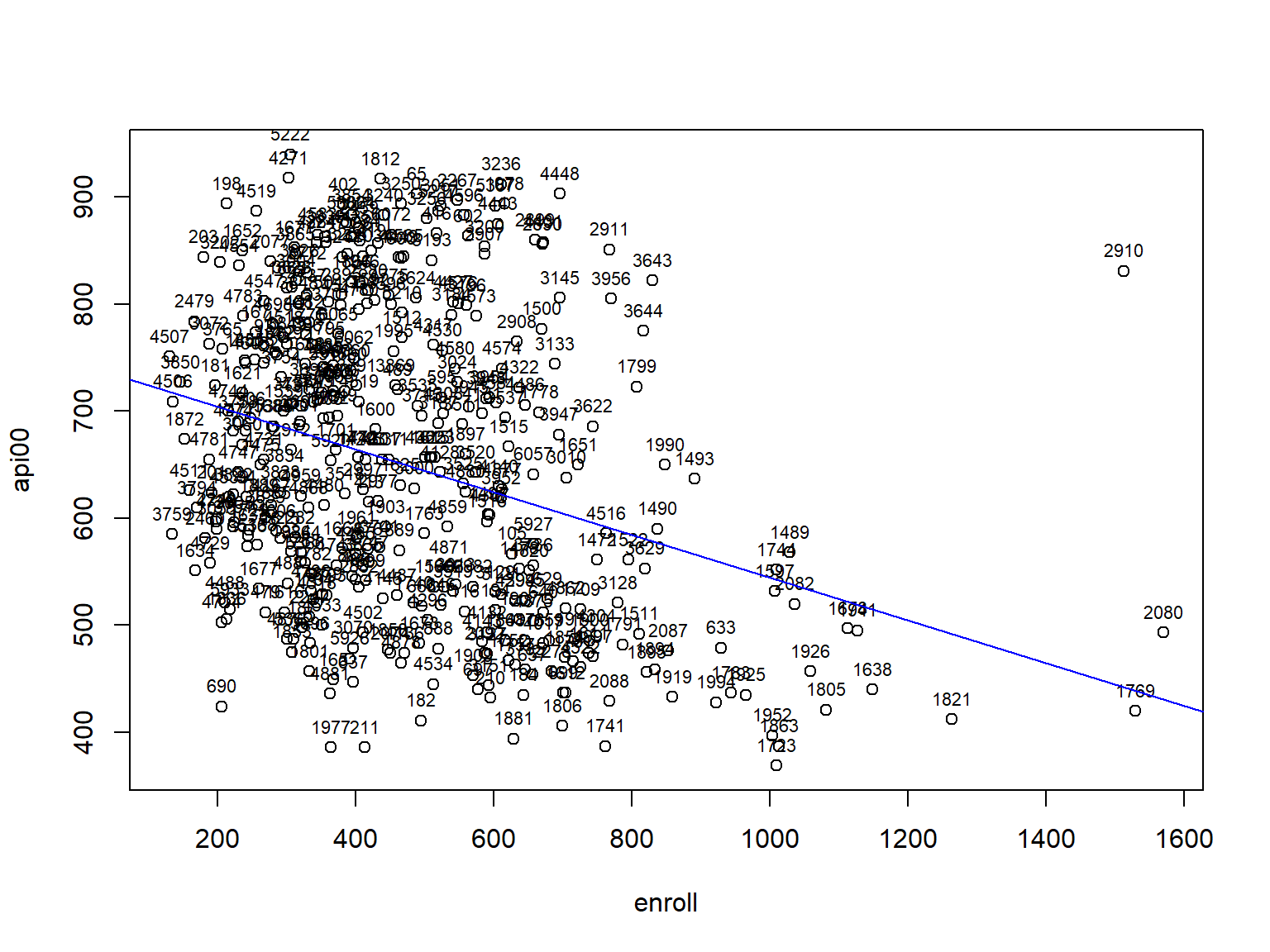##We can also use identify() to click on the points
# plot(api00 ~ enroll, data = d)
# abline(m1, col = "blue")
# identify(d$enroll, d$api00,labels = d$snum, col = "red") #Optional if someone likes to use ggplot library(ggplot2) ggplot(d, aes(x = enroll, y = api00)) + geom_point() + stat_smooth(method = "lm", col = "red")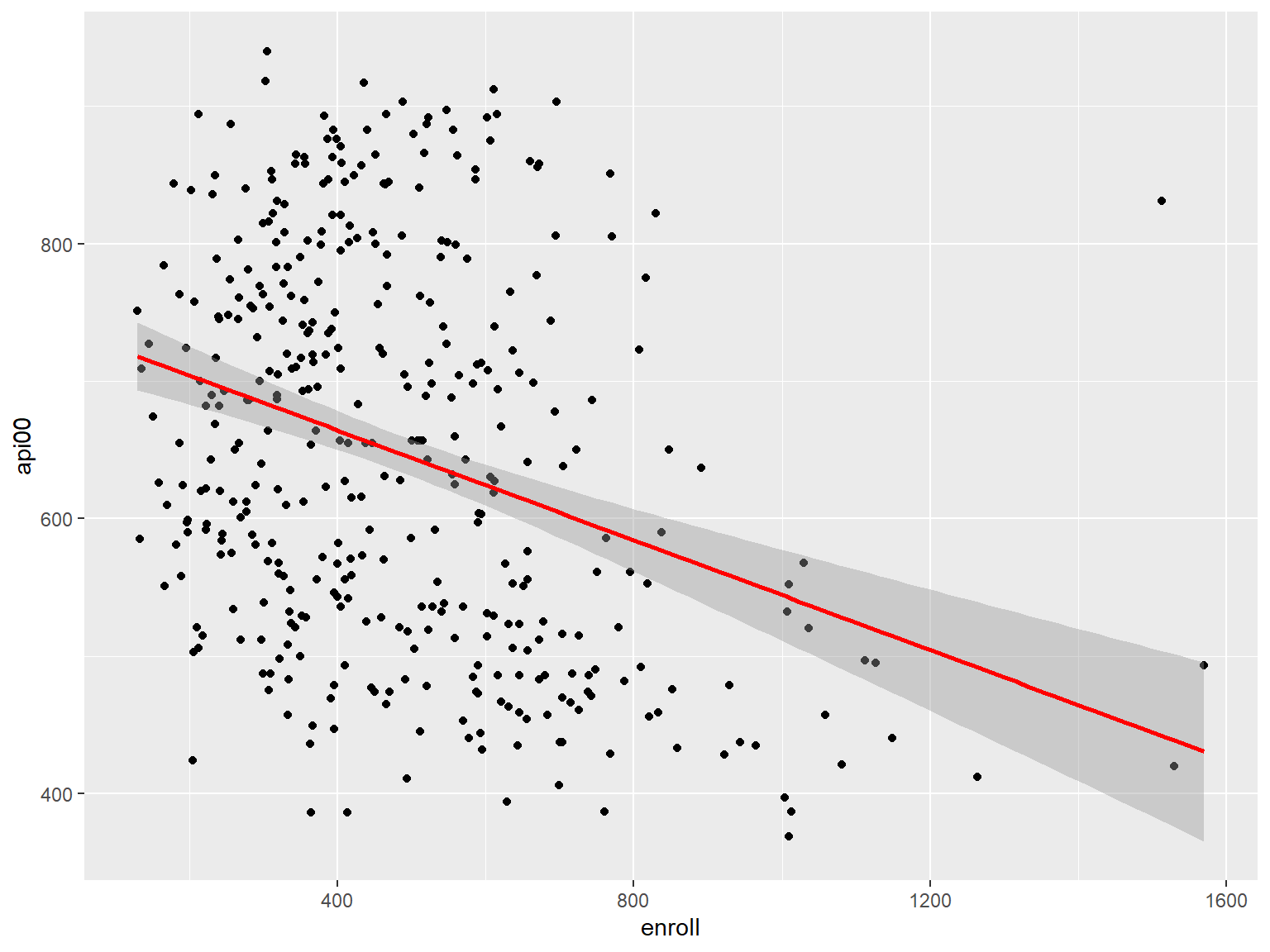# 2.9 Exercise 1 In the data set elemapi2v2 the variable full is the percentage teachers with full credential for each school. Run the regression model of api00 on full. Use this model to predict the mean of api00 for full equal to $$25\%$$, $$50\%$$, $$75\%$$, and $$90\%$$ Is the predicted value for full $$= 25\%$$ valid? # 3 Regression with categorical variable as predictor # 3.1 Factor and dummy variable • So far we have focused on regression analyses using continuous variables. However, it is possible to include categorical predictors in a regression analysis, but it requires some extra work in performing the analysis and extra work to properly interpreting the results. • For example in the elemapi2v2 data, yr_rnd and mealcat are categorical variables and we use them as predictor to model api00. • The Variable yr_rnd take value 0 for the school that is not year round and 1 for school that is year round school. • The variable meals is the percentage of students who are receiving state sponsored free meals and can be used as an indicator of poverty. This variable is broken into 3 categories (to make equally sized groups) creating the variable mealcat as follow: mealcat meals 1 0-46% free meals 2 47-80% free meals 3 81-100% free meals • R uses object class factor to store and manipulate categorical variables. The lm function automatically treat variable type character as factor, but it is always safer to change the variable’s class to factor before the analysis. • The function factor() is used to encode a variable (a vector) as a factor. #class of mealcat is integer class(d$mealcat) 
##  "integer"
#we transform variable meancat to a factor variable
d$mealcat_F <- factor(d$mealcat)
#now the class of mealcat_F is factor
str(d$mealcat_F) ## Factor w/ 3 levels "1","2","3": 2 3 3 3 3 1 1 1 1 1 ... #class of yr_rnd is integer class(d$yr_rnd) 
##  "integer"
#we transform variable yr_rnd to a factor variable
d$yr_rnd_F <- factor(d$yr_rnd)
#levels of factor variable yr_rnd_F
levels(d$yr_rnd_F) ##  "0" "1" #changing the levels to "Yes" and "No" levels(d$yr_rnd_F) <- c("No", "Yes")

Let’s look at frequency table of variables yr_rnd_F and mealcat_F

table(d$yr_rnd_F) #frequency table for yr_rnd_F ## ## No Yes ## 308 92 table(d$mealcat_F)   #frequency table for mealcat_F
##
##   1   2   3
## 131 132 137
• In R when we include a factor as a predictor to the model, the lm function by default generates dummy variables for each category of the factor. A dummy variable is a variable that takes values 0 and 1. If we are in that category the dummy variable is 1 and if we are not in that category the dummy variable is 0.

• The number of dummy variables is equal to the number of levels for the categorical variable minus 1. For example if we have 3 levels for the categorical variables, lm generates 2 dummy variables. If value for both dummy variable is 0 then we are in the third category. This third category is called the reference category.

• For example, factor yr_rnd_F with two levels of “Yes” and “No” (“No” is the reference category) is coded as only one dummy variable.

yr_rnd_F yr_rnd_FYes
“No” 0
“Yes” 1
• Factor mealcat_F with three levels of “1”, “2”, and “3” (“1” is the reference category) is coded as two dummy variables.

mealcat_F mealcat_F2 mealcat_F3
“1” 0 0
“2” 1 0
“3” 0 1

# 3.2 Regression of api00 on yr_rnd a two level categorical variable

• In the first example we use variable yr_rnd_F as independent variable and and api00 as response variable.
#regression of api00 with yr_rnd_F
m2 <- lm(api00 ~ yr_rnd_F, data = d)
#summary of the model
summary(m2)
##
## Call:
## lm(formula = api00 ~ yr_rnd_F, data = d)
##
## Residuals:
##      Min       1Q   Median       3Q      Max
## -273.539  -95.662    0.967  103.341  297.967
##
## Coefficients:
##             Estimate Std. Error t value Pr(>|t|)
## (Intercept)   684.54       7.14   95.88   <2e-16 ***
## yr_rnd_FYes  -160.51      14.89  -10.78   <2e-16 ***
## ---
## Signif. codes:  0 '***' 0.001 '**' 0.01 '*' 0.05 '.' 0.1 ' ' 1
##
## Residual standard error: 125.3 on 398 degrees of freedom
## Multiple R-squared:  0.226,  Adjusted R-squared:  0.2241
## F-statistic: 116.2 on 1 and 398 DF,  p-value: < 2.2e-16
#the first 6 rows of the model matrix
model.matrix(m2)[1:6,]
##   (Intercept) yr_rnd_FYes
## 1           1           0
## 2           1           0
## 3           1           0
## 4           1           0
## 5           1           0
## 6           1           0

Let’s write out the regression equation that this model implies.

$\text{api00} = 684.539 + (-160.5064) \times \text{yr_rnd_FYes}$ - As we have discussed before the dummy variable yr_rnd_FYes gets value 1 if yr_rnd_F is “Yes” and 0 if yr_rnd_F is “No”.

If a school is not a year-round school (i.e. yr_rnd_F is 0) the regression equation would simplify to

api00 = 684.539    + (-160.5064) x 0
api00 = 684.539

This indicates the mean of api00 given the school is not a year-round school is $$684.539$$.

If a school is a year-round school, the regression equation would simplify to

api00 = 684.539   +  (-160.5064) x 1
api00 = 524.0326

This indicates the mean of api00 given the school is not a year-round school is $$524.0326$$.

In general when we have a two level categorical variable the intercept is the expected outcome for the reference group and the slope of the other category is the difference of expected outcome of that group with the reference category.

• We can graph the observed values and the predicted values using the plot of api00 against original variable for yr_rnd, where it is an integer. Although yr_rnd only has 2 values, we can still draw a regression line showing the relationship between yr_rnd and api00.
#scatter plot api00 against yr_rnd
plot(api00 ~ yr_rnd, data = d)
abline(m2)  # add regression line to the plot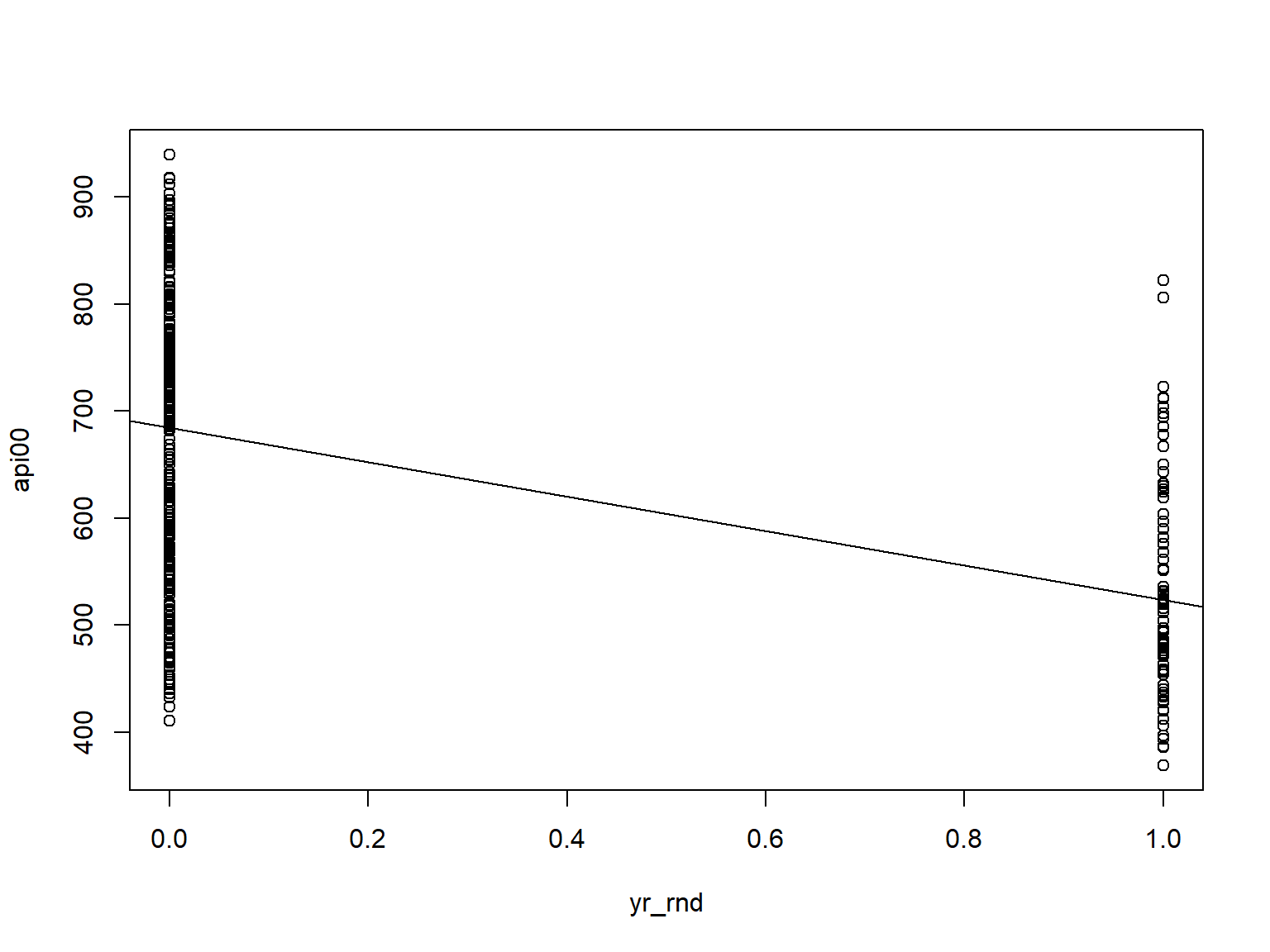# 3.3 Regression of api00 on mealcat, a three level categorical variable

In this example we would like to examine the relationship between the amount of poverty and api scores. We don’t have a measure of poverty, but we can use mealcat as a proxy for a measure of poverty. We have created a factor variable for mealcat named mealcat_F and we can use it to model regression of api00 with mealcat_F.

#regression model of api00 against categorical variable mealcat_F with 3 levels
m3 <- lm(api00 ~ mealcat_F, data = d)
summary(m3)
##
## Call:
## lm(formula = api00 ~ mealcat_F, data = d)
##
## Residuals:
##      Min       1Q   Median       3Q      Max
## -253.394  -47.883    0.282   52.282  185.620
##
## Coefficients:
##             Estimate Std. Error t value Pr(>|t|)
## (Intercept)  805.718      6.169  130.60   <2e-16 ***
## mealcat_F2  -166.324      8.708  -19.10   <2e-16 ***
## mealcat_F3  -301.338      8.629  -34.92   <2e-16 ***
## ---
## Signif. codes:  0 '***' 0.001 '**' 0.01 '*' 0.05 '.' 0.1 ' ' 1
##
## Residual standard error: 70.61 on 397 degrees of freedom
## Multiple R-squared:  0.7548, Adjusted R-squared:  0.7536
## F-statistic: 611.1 on 2 and 397 DF,  p-value: < 2.2e-16
• As we have discussed before, the lm function creates two dummy variables for three level categorical variable mealcat_F. The above model is basically a multiple regression model with two predictor variables. In the next section we will discuss multiple regression model in more detail.

• The interpretation of the coefficients is much like that for the binary variables. Group 1 is the omitted group, so Intercept is the mean for group 1. The coefficient for mealcat_F2 is the mean for group 2 minus the mean of the omitted group (group 1). And the coefficient for mealcat_F3 is the mean of group 3 minus the mean of group 1. We can verify this by calculating the means of api00 for the groups of mealcat_F.

#aggregate the mean of api00 for each category in mealcat_F
aggregate(api00 ~ mealcat_F, FUN=mean, data = d)
##   mealcat_F    api00
## 1         1 805.7176
## 2         2 639.3939
## 3         3 504.3796
• Based on these results, we can say that the three groups differ in their api00 scores. In particular group2 is significantly different from group1 and group 3 is significantly different from group 1.

• The F test at the last line give a test for the overall differences of api00 among the three groups of mealcat_F.

• We can also change the reference group by rearranging the levels of factor mealcat_F

#relevel factor mealcat_F and make group "3" as the reference group
d$mealcat_F <- relevel(d$mealcat_F, ref = "3")
m4 <- lm(api00 ~ mealcat_F, data = d)
summary(m4)
##
## Call:
## lm(formula = api00 ~ mealcat_F, data = d)
##
## Residuals:
##      Min       1Q   Median       3Q      Max
## -253.394  -47.883    0.282   52.282  185.620
##
## Coefficients:
##             Estimate Std. Error t value Pr(>|t|)
## (Intercept)  504.380      6.033   83.61   <2e-16 ***
## mealcat_F1   301.338      8.629   34.92   <2e-16 ***
## mealcat_F2   135.014      8.612   15.68   <2e-16 ***
## ---
## Signif. codes:  0 '***' 0.001 '**' 0.01 '*' 0.05 '.' 0.1 ' ' 1
##
## Residual standard error: 70.61 on 397 degrees of freedom
## Multiple R-squared:  0.7548, Adjusted R-squared:  0.7536
## F-statistic: 611.1 on 2 and 397 DF,  p-value: < 2.2e-16
• With group 3 omitted, the intercept now is the mean of api00 for group mealcat_F = 3, and mealcat_F1 is the difference of the mean of api00 for mealcat_F = 1 and the mean of api00 for mealcat_F = 3, and mealcat_F2 is the difference of the mean of api00 for mealcat_F = 2 and the mean of api00 for mealcat_F = 3.

# 3.4 regression analysis with a single dummy variable and t-test (Optional)

• Note that if you plot api00 against factor yr_rnd_F you will get a side by side boxplot.
#scatter plot api00 against yr_rnd_F
plot(api00 ~ yr_rnd_F, data = d)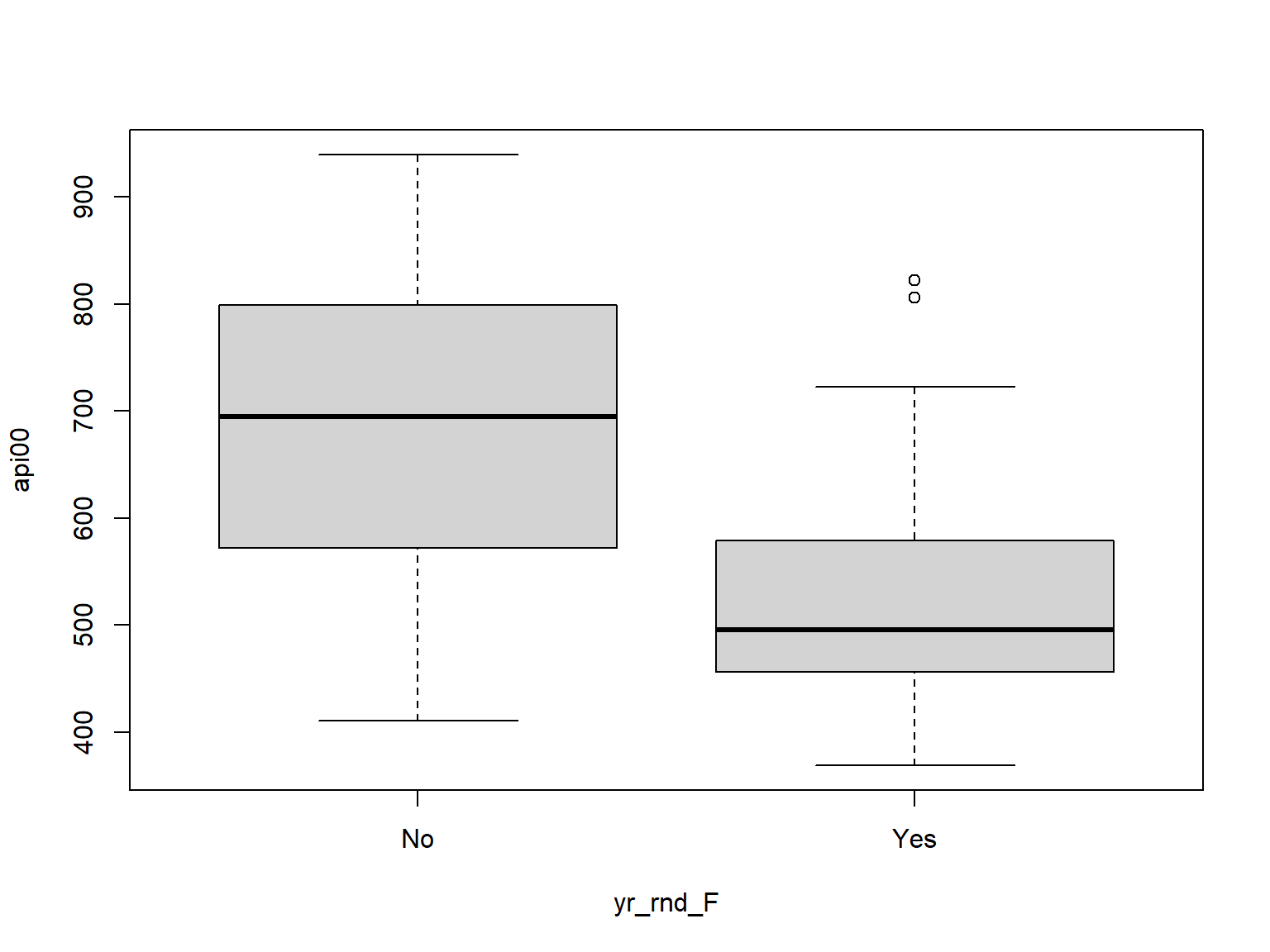• Let’s compare these predicted values to the mean api00 scores for the year-round and non-year-round schools.
# mean of api00 when yr_rnd_F is at level "0". school is not year round
mean(d$api00[d$yr_rnd_F == "0"])
##  NaN
# mean of api00 when yr_rnd_F is at level "1". school is year round
mean(d$api00[d$yr_rnd_F == "1"])
##  NaN
• You can use function aggregate to do the above.
#using aggregate to find the mean for each group of year school round
aggregate(api00 ~ yr_rnd_F, FUN=mean, data = d)
##   yr_rnd_F    api00
## 1       No 684.5390
## 2      Yes 524.0326
• As you see, the regression equation predicts that the value of api00 will be the mean value, depending on whether a school is a year round school or non-year round school.

• Let’s relate these predicted values back to the regression equation. For the non-year-round schools, their mean is the same as the intercept (684.539). The coefficient for yr_rnd is the amount we need to add to get the mean for the year-round schools, i.e., we need to add -160.5064 to get 524.0326, the mean for the non year-round schools. In other words, Byr_rnd is the mean api00 score for the year-round schools minus the mean api00 score for the non year-round schools, i.e., mean(year-round) - mean(non year-round).

• It may be surprising to note that this regression analysis with a single dummy variable is the same as doing a t-test comparing the mean api00 for the year-round schools with the non year-round schools (see below). You can see that the t value below is the same as the t value for yr_rnd in the regression above.

#t test for equality of mean of api00 for two group of year round and not year round
#with equal variance assumption
t.test(api00 ~ yr_rnd_F, data = d, var.equal = TRUE)
##
##  Two Sample t-test
##
## data:  api00 by yr_rnd_F
## t = 10.782, df = 398, p-value < 2.2e-16
## alternative hypothesis: true difference in means between group No and group Yes is not equal to 0
## 95 percent confidence interval:
##  131.2390 189.7737
## sample estimates:
##  mean in group No mean in group Yes
##          684.5390          524.0326

Since a t-test is the same as doing an anova, we can get the same results using the anova command as well.

#anova table
anova(m2)
## Analysis of Variance Table
##
## Response: api00
##            Df  Sum Sq Mean Sq F value    Pr(>F)
## yr_rnd_F    1 1825001 1825001  116.24 < 2.2e-16 ***
## Residuals 398 6248671   15700
## ---
## Signif. codes:  0 '***' 0.001 '**' 0.01 '*' 0.05 '.' 0.1 ' ' 1

If we square the t-value from the t-test, we get the same value as the F-value from the anova.

#square of t value from the t-test is the same as F value from anova
10.7815 ^ 2
##  116.2407

# 3.5 Exercise 2

In the data set elemapi2v2 the variable collcat is categorized of the percentage of students with parent attended in some collage for each school. Run the regression model of api00 on collcat as a categorical variable. What is the expected average api00 score for a school with collcat in the 3rd category?

# 4.1 Adding more predictors to a simple regression model

Now, let’s look at an example of multiple regression, in which we have one outcome (dependent) variable and multiple predictors.

The percentage of variability of api00 explained by variable enroll was only 10.12%. In order to explain more variation, we can add more predictors. In R we can do this by adding variables with + to the formula of our lm() function. We add meals, the percentage of students who get full meals as an indicator of socioeconomic status, and full, the percentage of teachers with full credentials, to our model.

#multiple regression model of DV api00 and IVs enroll, meals, and full
m5 <- lm(api00 ~  enroll + meals + full, data = d)
summary(m5)   #summary of model m5
##
## Call:
## lm(formula = api00 ~ enroll + meals + full, data = d)
##
## Residuals:
##      Min       1Q   Median       3Q      Max
## -181.721  -40.802    1.129   39.983  158.774
##
## Coefficients:
##              Estimate Std. Error t value Pr(>|t|)
## (Intercept) 801.82983   26.42660  30.342  < 2e-16 ***
## enroll       -0.05146    0.01384  -3.719 0.000229 ***
## meals        -3.65973    0.10880 -33.639  < 2e-16 ***
## full          1.08109    0.23945   4.515 8.37e-06 ***
## ---
## Signif. codes:  0 '***' 0.001 '**' 0.01 '*' 0.05 '.' 0.1 ' ' 1
##
## Residual standard error: 58.73 on 396 degrees of freedom
## Multiple R-squared:  0.8308, Adjusted R-squared:  0.8295
## F-statistic: 648.2 on 3 and 396 DF,  p-value: < 2.2e-16
anova(m5)     #anova table of model m5 
## Analysis of Variance Table
##
## Response: api00
##            Df  Sum Sq Mean Sq  F value    Pr(>F)
## enroll      1  817326  817326  236.947 < 2.2e-16 ***
## meals       1 5820066 5820066 1687.263 < 2.2e-16 ***
## full        1   70313   70313   20.384 8.369e-06 ***
## Residuals 396 1365967    3449
## ---
## Signif. codes:  0 '***' 0.001 '**' 0.01 '*' 0.05 '.' 0.1 ' ' 1
• We see that, based on the F-test, the overall model is a significant improvement in fit compared to the intercept-only model. Also, all of the tests of the coefficients against the null value of zero are significant.

• The R-square is 0.8308, meaning that approximately 83% of the variability of api00 is accounted for by the variables in the model. The adjusted R-square shows after taking the account of number of predictors in the model R_square is still about 0.83.

• The coefficients for each of the variables indicates the amount of change one could expect in api00 given a one-unit increase in the value of that variable, given that all other variables in the model are held constant. For example, consider the variable meals. We would expect a decrease of about 3.66 in the api00 score for every one unit increase in percent free meals, assuming that all other variables in the model are held constant.

• We see quite a difference in the coefficient of variable enroll compared to the simple linear regression. Before the coefficient for variable enroll was -.1999 and now it is -0.05146.

• The ANOVA table shows the sum of squares explained by adding each variable sequentially to the model, or equivalently, the amount of sum of square residuals reduced by each additional variable.

For example variable enroll reduces the total error (RSS) by 817326. By adding variable meals we reduce additional 5820066 from the residual sum of squares and by adding variable full we reduce the error by 70313. Finally we have 1365967 left as unexplained error. The total sum of squares (TSS) is the sum of all of the sums of squares added together. To get the total sum of square of variable api00 we can multiply its’ variance by $$(n-1)$$.

sum(anova(m5)$Sum) #sum of RSS and SSreg ##  8073672 (400 - 1) * var(d$api00)    #Total sum of squares 
##  8073672

# 4.2 Standardized regression coefficients

Some researchers are interested in comparing the relative strength of the various predictors within the model. Since each variable is typically measured in different units, we cannot compare coefficients to one another. To address this problem we use standardized regression coefficients, which can be obtain by transforming the outcome and predictor variables all to their standardized scores, also called z-scores, before running the regression.

In R we use function scale() to do this for each variable.

#Standardized regression model
m5.sd <- lm(scale(api00) ~  scale(enroll) + scale(meals) + scale(full), data = d)
summary(m5.sd) #coefficients are standardized
##
## Call:
## lm(formula = scale(api00) ~ scale(enroll) + scale(meals) + scale(full),
##     data = d)
##
## Residuals:
##      Min       1Q   Median       3Q      Max
## -1.27749 -0.28683  0.00793  0.28108  1.11617
##
## Coefficients:
##                 Estimate Std. Error t value Pr(>|t|)
## (Intercept)    4.454e-16  2.064e-02   0.000 1.000000
## scale(enroll) -8.191e-02  2.203e-02  -3.719 0.000229 ***
## scale(meals)  -8.210e-01  2.441e-02 -33.639  < 2e-16 ***
## scale(full)    1.136e-01  2.517e-02   4.515 8.37e-06 ***
## ---
## Signif. codes:  0 '***' 0.001 '**' 0.01 '*' 0.05 '.' 0.1 ' ' 1
##
## Residual standard error: 0.4129 on 396 degrees of freedom
## Multiple R-squared:  0.8308, Adjusted R-squared:  0.8295
## F-statistic: 648.2 on 3 and 396 DF,  p-value: < 2.2e-16

In the standardized regression coefficients summary we see that the intercept is zero and all t-statistics (p-values) for the other coefficients are exactly the same as the original model.

Because the coefficients are all in the same standardized units, standard deviations, you can compare these coefficients to assess the relative strength of each of the predictors. In this example, meals has the largest Beta coefficient, -0.821.

Thus, a one standard deviation increase in meals leads to a 0.821 standard deviation decrease in predicted api00, with the other variables held constant.

# 5.1 Regression model with interaction between two continuous predictors

• For the first model with interaction we use regression model of api00 with enroll and meals including interaction between between enroll and meals.
#multiple regression model of DV api00 and IVs enroll, meals, and enroll:meals
m6 <- lm(api00 ~  enroll + meals + enroll:meals , data = d)
summary(m6)   #summary of model m6
##
## Call:
## lm(formula = api00 ~ enroll + meals + enroll:meals, data = d)
##
## Residuals:
##      Min       1Q   Median       3Q      Max
## -211.186  -38.834   -1.137   38.997  163.713
##
## Coefficients:
##                Estimate Std. Error t value Pr(>|t|)
## (Intercept)   8.833e+02  1.665e+01  53.034   <2e-16 ***
## enroll        8.835e-04  3.362e-02   0.026   0.9790
## meals        -3.425e+00  2.344e-01 -14.614   <2e-16 ***
## enroll:meals -9.537e-04  4.292e-04  -2.222   0.0269 *
## ---
## Signif. codes:  0 '***' 0.001 '**' 0.01 '*' 0.05 '.' 0.1 ' ' 1
##
## Residual standard error: 59.85 on 396 degrees of freedom
## Multiple R-squared:  0.8243, Adjusted R-squared:  0.823
## F-statistic: 619.3 on 3 and 396 DF,  p-value: < 2.2e-16
• The intercept interpreted as before and is the expected api00 when both enroll and meals are set to zero.

• The coefficient of enroll is interpreted as increase of the mean of api00 by $$0.0008835$$ for one unit increase of number of enrollment given the value of meals is zero.

• The coefficient of meals is interpreted as average decrease of the mean of api00 by $$3.425$$ for one unit increase of meals (one percentage increase) given the value of enroll is zero.

• Adding interaction term means that the effect of enroll and meals is no longer constant for different values of the other predictor.

• The interaction term can be interpreted in two ways.

• If we use enroll as moderator variable then we can say that the effect of meals changes by $$-0.0009537$$ for one unit increase of enroll.

• If we use meals as moderator variable then we can say that the effect of enroll changes by $$-0.0009537$$ for one unit increase of meals.

# 5.2 Regression model with interaction between two categorical predictors

• For the model with interaction between two categorical predictors, we use regression model of api00 with yr_rnd_F and mealcat_F including interaction between between yr_rnd_F and mealcat_F. In the model formula, we can use api00 ~ yr_rnd_F * mealcat_F to include main terms and interaction term together.
#multiple regression model of DV api00 and IVs enroll, meals, and enroll:meals
m7 <- lm(api00 ~  yr_rnd_F * mealcat_F , data = d)
summary(m7)   #summary of model m7
##
## Call:
## lm(formula = api00 ~ yr_rnd_F * mealcat_F, data = d)
##
## Residuals:
##      Min       1Q   Median       3Q      Max
## -207.533  -50.764   -1.843   48.874  179.000
##
## Coefficients:
##                        Estimate Std. Error t value Pr(>|t|)
## (Intercept)             521.493      8.414  61.978  < 2e-16 ***
## yr_rnd_FYes             -33.493     11.771  -2.845  0.00467 **
## mealcat_F1              288.193     10.443  27.597  < 2e-16 ***
## mealcat_F2              123.781     10.552  11.731  < 2e-16 ***
## yr_rnd_FYes:mealcat_F1  -40.764     29.231  -1.395  0.16394
## yr_rnd_FYes:mealcat_F2  -18.248     22.256  -0.820  0.41278
## ---
## Signif. codes:  0 '***' 0.001 '**' 0.01 '*' 0.05 '.' 0.1 ' ' 1
##
## Residual standard error: 68.87 on 394 degrees of freedom
## Multiple R-squared:  0.7685, Adjusted R-squared:  0.7656
## F-statistic: 261.6 on 5 and 394 DF,  p-value: < 2.2e-16
• The intercept interpreted as the expected api00 when both yr_rnd_F and mealcat_F are at their reference level. Here, it is the expected api00 given school is not year round and the meals category is at level “3”.

• The coefficient of yr_rnd_FYes is the difference of the expected api00 for year round school with school is not year round when meals category is at level “3”

• The coefficient of mealcat_F1 is the difference of the expected api00 between mealcat_F at level “1” and mealcat_F at level “3” given school is not year round.

• The coefficient of mealcat_F2 is the difference of the expected api00 between mealcat_F at level “2” and mealcat_F at level “3” given school is not year round.

• The interaction terms can be interpreted in two ways. Here we only interpret it in one way:

• The coefficient of yr_rnd_FYes:mealcat_F1 is the expected difference api00 for year round school with school is not year round if the meals category is “1” minus the expected difference api00 for year round school with school is not year round if the meals category is “3”.

• The coefficient of yr_rnd_FYes:mealcat_F2 is the expected api00 for year round school with school is not year round if the meals category is “2” minus the expected api00 for year round school with school is not year round if the meals category is “3”.

• For example the expected mean of api00 for a school that is year round with the mealcat equal to “2” is:

$521.493 + (-33.493) + 123.781 + (-18.248) = 593.533$

The expected mean of api00 for a school that is not year round with the mealcat equals to “2” is:

$521.493 + 123.781 = 645.274$ Thus, the expected difference api00 for year round school with school is not year round if the meals category equals to “2” is:

$593.533 - 645.274 = -51.741$

The difference of the expected api00 for year round school with school is not year round when meals category is at level “3 is the coefficient of yr_rnd_FYes $$= -33.493$$

If we calculate the difference between the above differences we get:

$-51.741 - (-33.493) = -18.248$

which is the coefficient for yr_rnd_FYes:mealcat_F2.

• If we need to test overall effect of interaction terms (i.e. simultaneously test both interaction coefficients equal to zero) we can use likelihood ratio F test using anova function. To do so, we run a model without interaction terms and use anova function to test the difference between two models.
#running the model without interaction terms
m0 <- lm(api00 ~  yr_rnd_F + mealcat_F , data = d)
#run F-test using anova function
anova(m0, m7)
## Analysis of Variance Table
##
## Model 1: api00 ~ yr_rnd_F + mealcat_F
## Model 2: api00 ~ yr_rnd_F * mealcat_F
##   Res.Df     RSS Df Sum of Sq      F Pr(>F)
## 1    396 1879528
## 2    394 1868944  2     10584 1.1156 0.3288

The F test means that we do not have enough evidence to reject the base model m0 and therefore, the effect yr_rnd unlikely change for different levels of mealcat.

# 5.3 Regression model with interaction between a continuous predictor and a categorical predictor

• For the model with interaction between two categorical predictors, we use regression model of api00 with yr_rnd_F and enroll including interaction between between yr_rnd_F and enroll. In the model formula, we can use api00 ~ yr_rnd_F * enroll to include main terms and interaction term together.
#multiple regression model of DV api00 and IVs enroll, meals, and enroll:meals
m8 <- lm(api00 ~  yr_rnd_F * enroll , data = d)
summary(m8)   #summary of model m8
##
## Call:
## lm(formula = api00 ~ yr_rnd_F * enroll, data = d)
##
## Residuals:
##      Min       1Q   Median       3Q      Max
## -274.043  -94.781    0.417   97.666  309.573
##
## Coefficients:
##                      Estimate Std. Error t value Pr(>|t|)
## (Intercept)        682.068556  18.797436  36.285   <2e-16 ***
## yr_rnd_FYes        -74.858236  48.224281  -1.552   0.1214
## enroll               0.006021   0.042396   0.142   0.8871
## yr_rnd_FYes:enroll  -0.120218   0.072075  -1.668   0.0961 .
## ---
## Signif. codes:  0 '***' 0.001 '**' 0.01 '*' 0.05 '.' 0.1 ' ' 1
##
## Residual standard error: 125 on 396 degrees of freedom
## Multiple R-squared:  0.2335, Adjusted R-squared:  0.2277
## F-statistic: 40.21 on 3 and 396 DF,  p-value: < 2.2e-16
• The intercept interpreted as before and is the expected api00 when enroll is zero and the school is not year round.

• The coefficient of enroll is interpreted as increase of the mean of api00 by $$0.006021$$ for one unit increase of number of enrollment given the school is not year round.

• The coefficient of yr_rnd_FYes is interpreted as the difference between the expected api00 for year round school and not year round school when given the value of enroll is zero.

• The interaction term can be interpreted as:

• The change of the effect of enroll to the expected mean api00 when the school is year round to when the school is not year round.

# 5.4 Ploting the interaction between a continuous predictor and a categorical predictor

• Sometimes it is a good idea to plot the predicted values for different levels of predictors to visualize the interaction.

We use function predict for a new data to plot this interaction.

#First get the range of continuous predictor
range(d$enroll) ##  130 1570 #make a sequence of number of enroll from range of enroll 130-1570 increment by 5 new.enroll <- seq(130, 1570, 5) #Because we have two levels of yr_rnd_F we repeat enroll two times. new.yr_rnd <- rep(levels(d$yr_rnd_F), each = length(new.enroll))
#create new data
new.data.plot <- data.frame(enroll = rep(new.enroll, times = 2),  yr_rnd_F = new.yr_rnd)
#predict api00
new.data.plot$predicted_api00 <- predict(m8, newdata = new.data.plot) #I use ggplot to plot library(ggplot2) ggplot(new.data.plot, aes(x = enroll, y = predicted_api00, colour = yr_rnd_F)) + geom_line(lwd=1.2)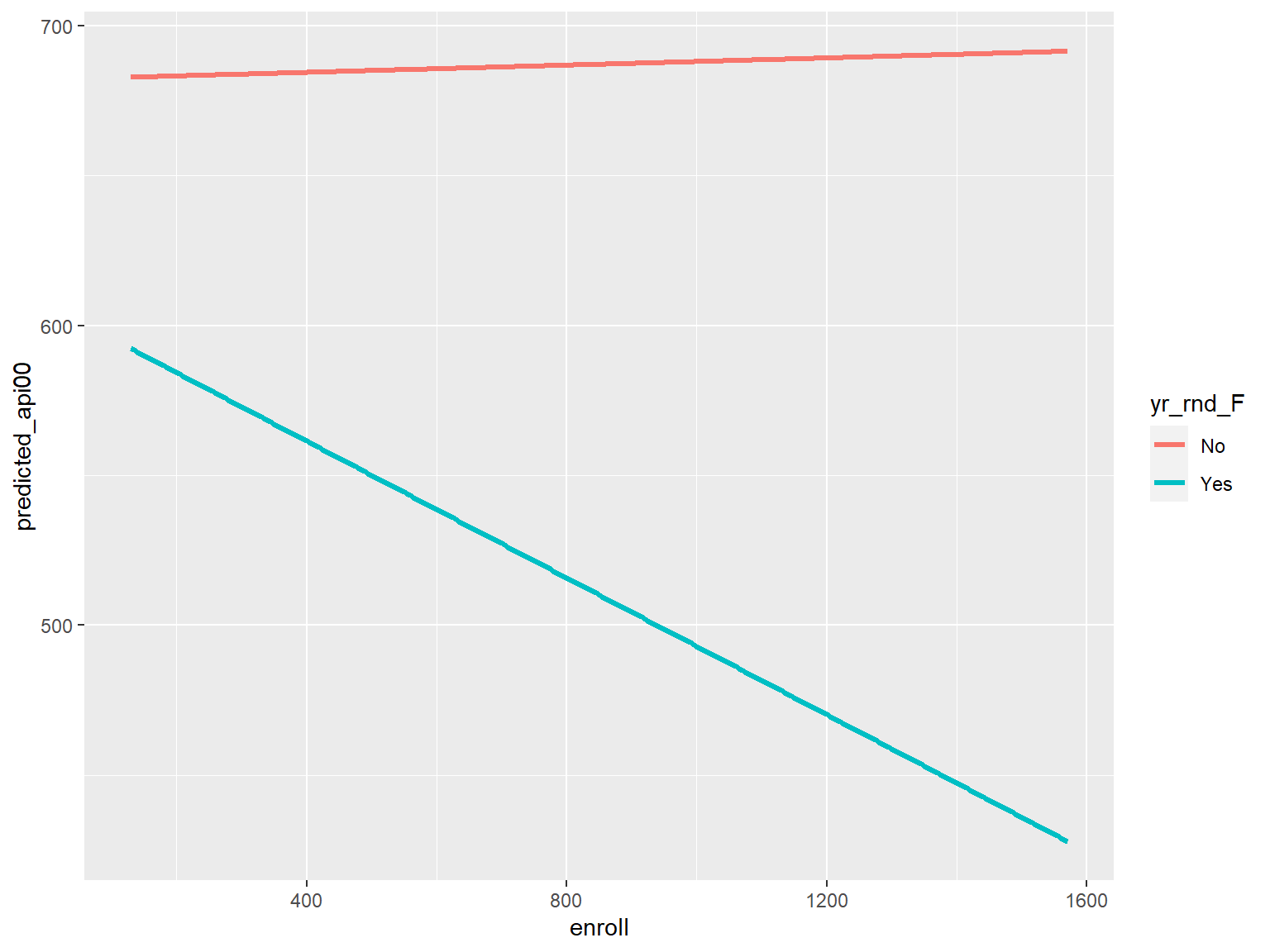# 5.5 Summary • In this part we have discussed the basics of how to perform simple and multiple regressions in R, the basics of interpreting output, as well as some related commands. There are more results that we can extract from the regression model and we have to skip them in this workshop. • We also can have a model with three way interactions which we do not have time to discuss in this workshop. • If the relationship between a predictor variable and the outcome is not linear we may be able to use transformation to fit the linear regression model. The simplest transformation is quadratic form. • We have not covered model selection which is very important in real data analysis where we have many predictors. • The next part we are going into a more thorough discussion of the assumptions of linear regression and how you can use R to assess these assumptions for your data. In particular, the next part will address the following issues. • Checking for points that exert undue influence on the coefficients • Checking for constant error variance (homoscedasticity) • Checking for linear relationships • Checking normality of residuals • Checking for multicollinearity # 6 Regression Diagnostics # 6.1 Introduction In the previous part, we learned how to do ordinary linear regression with R. Without verifying that the data have met the assumptions underlying OLS regression, results of regression analysis may be misleading. Here will explore how you can use R to check on how well your data meet the assumptions of OLS regression. In particular, we will consider the following assumptions. • Homogeneity of variance (homoscedasticity): The error variance should be constant • Linearity: the relationships between the predictors and the outcome variable should be linear • Independence: The errors associated with one observation are not correlated with the errors of any other observation • Normality: the errors should be normally distributed. Technically normality is necessary only for hypothesis tests to be valid. • Model specification: The model should be properly specified (including all relevant variables, and excluding irrelevant variables) Additionally, there are issues that can arise during the analysis that, while strictly speaking are not assumptions of regression, are none the less, of great concern to data analysts. • Influence: individual observations that exert undue influence on the coefficients • Collinearity: predictors that are highly collinear, i.e., linearly related, can cause problems in estimating the regression coefficients. Many graphical methods and numerical tests have been developed over the years for regression diagnostics. R has many of these methods in stats package which is already installed and loaded in R. There are some other tools in different packages that we can use by installing and loading those packages in our R environment. • Packages that we use in this part are: "car", "alr4", and "faraway". • To install a package in RStudio we can search for the package in the packages tab and install it or we can use R command install.packages("package name"). For example: install.packages("car") • We need to install a package only one time and after we close RStudio the package will exist in our local computer library. • To load a package in RStudio environment we use library(package name) or we can check the box for that package in the packages tab. • Every time we close an RSudio session and open it again we need to load the package into RStudio environment. # install packages for Regression Diagnostics #install.packages("car") #install.packages("alr4") #install.packages("faraway") #loading packages into working environment library(car) library(alr4) library(faraway) # 6.2 Unusual and Influential data A single observation that is substantially different from all other observations can make a large difference in the results of your regression analysis. If a single observation (or small group of observations) substantially changes your results, you would want to know about this and investigate further. There are three ways that an observation can be unusual. • Outliers: In linear regression, an outlier is an observation with large residual. In other words, it is an observation whose dependent-variable value is unusual given its values on the predictor variables. An outlier may indicate a sample peculiarity or may indicate a data entry error or other problem. • Leverage: An observation with an extreme value on a predictor variable is called a point with high leverage. Leverage is a measure of how far an observation deviates from the mean of that variable. These leverage points can have an effect on the estimate of regression coefficients. • Influence: An observation is said to be influential if removing the observation substantially changes the estimate of coefficients. Influence can be thought of as the product of leverage and outlierness. We use the model m2 in our last multiple regression model. In that model we predicted api00 by independent variables enroll, meals, and full. #multiple regression model of DV api00 and DVs enroll, meals, and full m2 <- lm(api00 ~ enroll + meals + full, data = d) Let’s make individual graphs of api00 with enroll and meals and full so we can get a better view of these scatter plots. scatterplotMatrix(~ api00 + enroll + meals + full, data = d)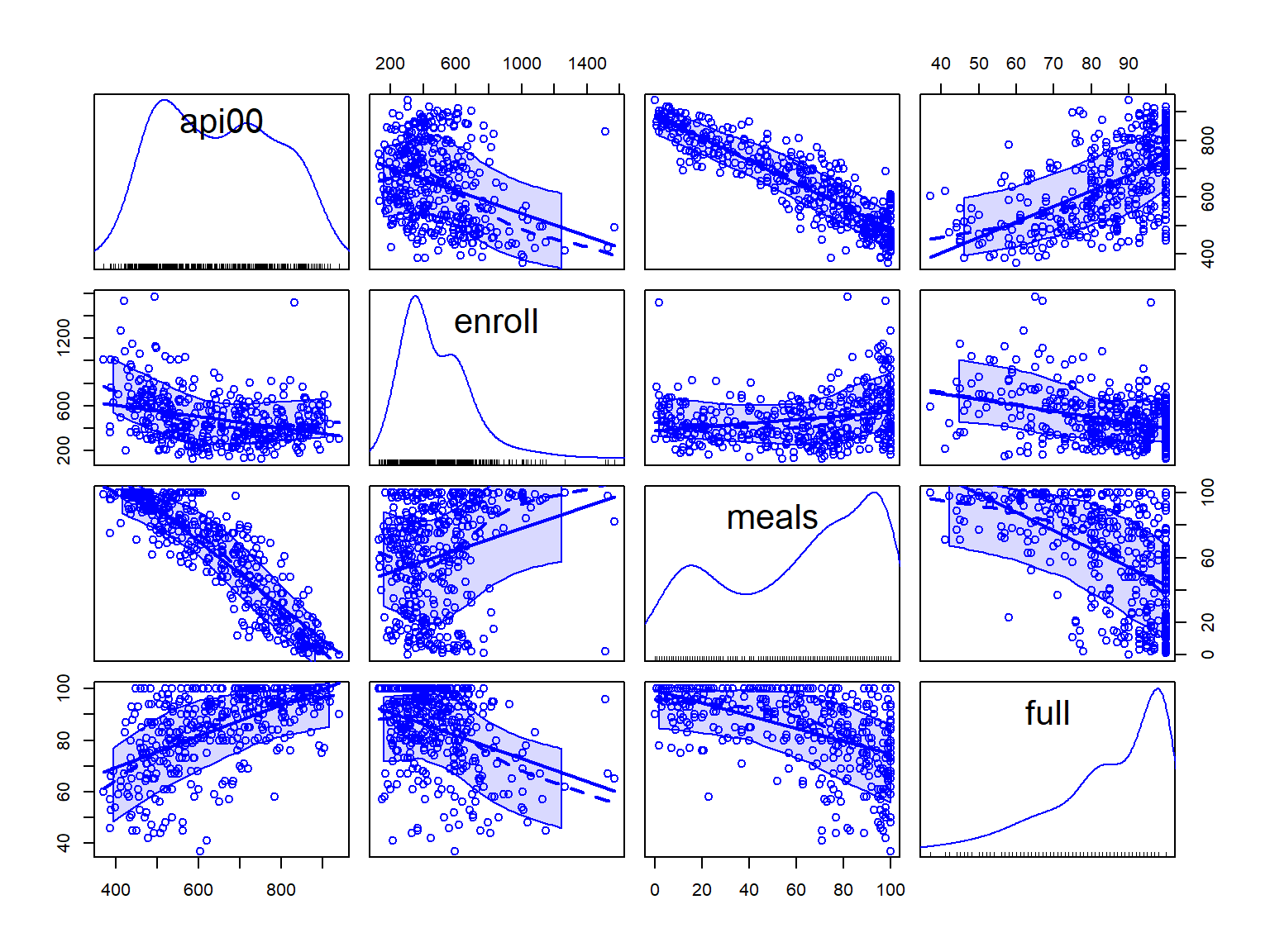The graphs of api00 with other variables show some potential problems. In every plot, we see one or more data point that is far away from the rest of the data points. We will go over some graphical methods to identify influential points and potential outliers. We are not going to discuss and justify underlying methods and formulas here. Studentized residuals can be used to identify outliers. In R we use rstandard() function to compute Studentized residuals. res.std <- rstandard(m2) #studentized residuals stored in vector res.std #plot Standardized residual in y axis. X axis will be the index or row names plot(res.std, ylab="Standardized Residual", ylim=c(-3.5,3.5)) #add horizontal lines 3 and -3 to identify extreme values abline(h =c(-3,0,3), lty = 2) #find out which data point is outside of 3 standard deviation cut-off #index is row numbers of those point index <- which(res.std > 3 | res.std < -3) #add School name next to points that have extreme value text(index-20, res.std[index] , labels = d$snum[index])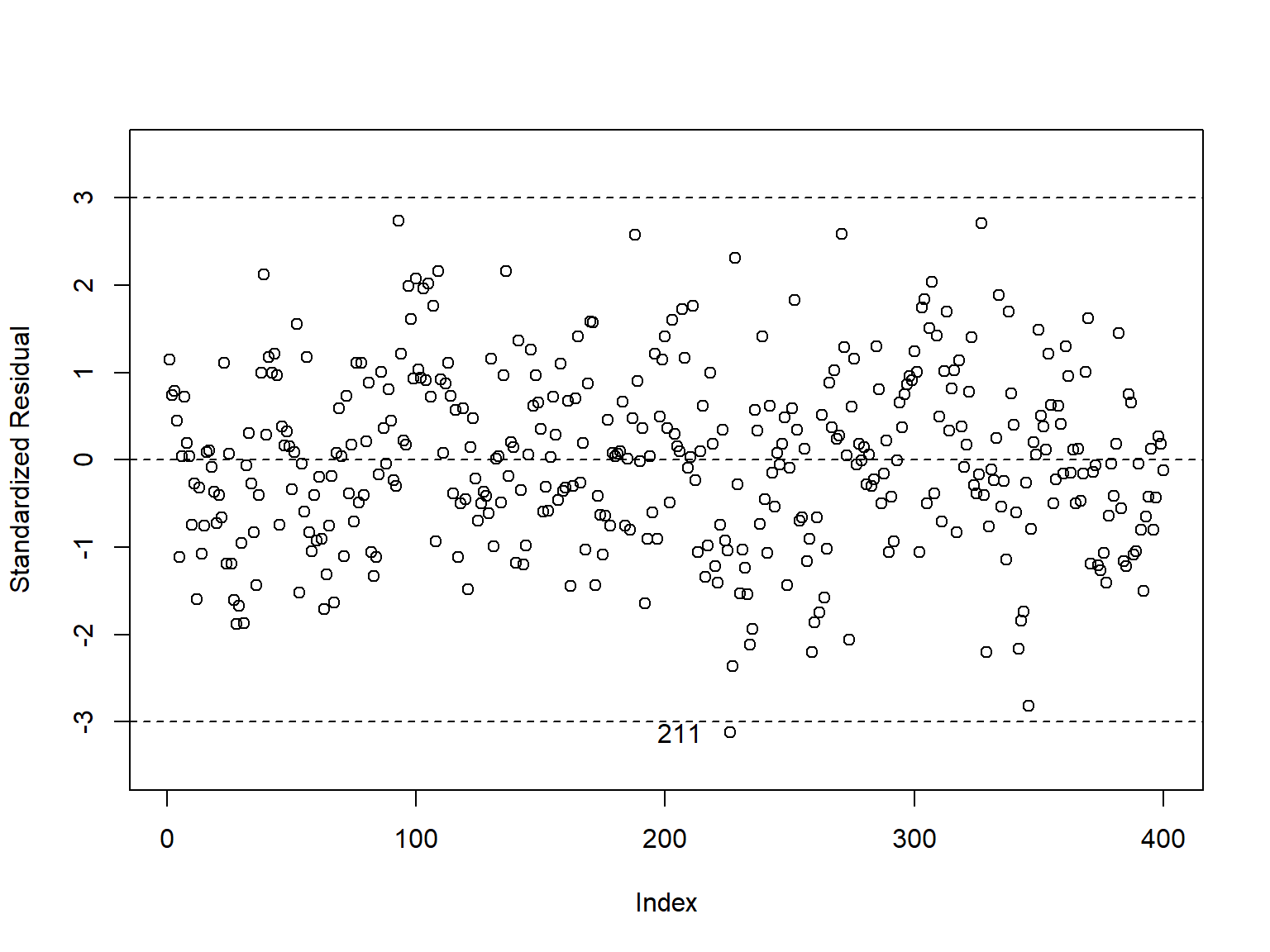#print row number of values that are out of bounds
print(index)
## 226
## 226
#print school names of points that are out of bounds
print(d$snum[index]) ##  211 We should pay attention to studentized residuals that exceed +2 or -2, and get even more concerned about residuals that exceed +2.5 or -2.5 and even yet more concerned about residuals that exceed +3 or -3. These results show that school number 211 is the most worrisome observation. Note: To get a t-statistics we use the externally Studentized residuals by excluding each point from the data to calculate standard deviation of residuals. In package "car" we can use function rstudent(model) to get the externally Studentized residuals. We can run a test statistic to test if we have outlier using function outlierTest(m2) in the package "car". #Bonferroni p-values for testing outliner outlierTest(m2) ## No Studentized residuals with Bonferroni p < 0.05 ## Largest |rstudent|: ## rstudent unadjusted p-value Bonferroni p ## 226 -3.151186 0.00175 0.70001 This is a test of hypothesis that we do not have outlier vs. at least we have one outlier. Now let’s look at the leverage’s to identify observations that will have potential great influence on regression coefficient estimates. To find points with high leverage is to use half normal plot in the package "faraway". First we need to compute diagonal of hat matrix as a measure for leverage #a vector containing the diagonal of the 'hat' matrix h <- influence(m2)$hat
#half normal plot of leverage from package faraway
halfnorm(influence(m2)$hat, ylab = "leverage")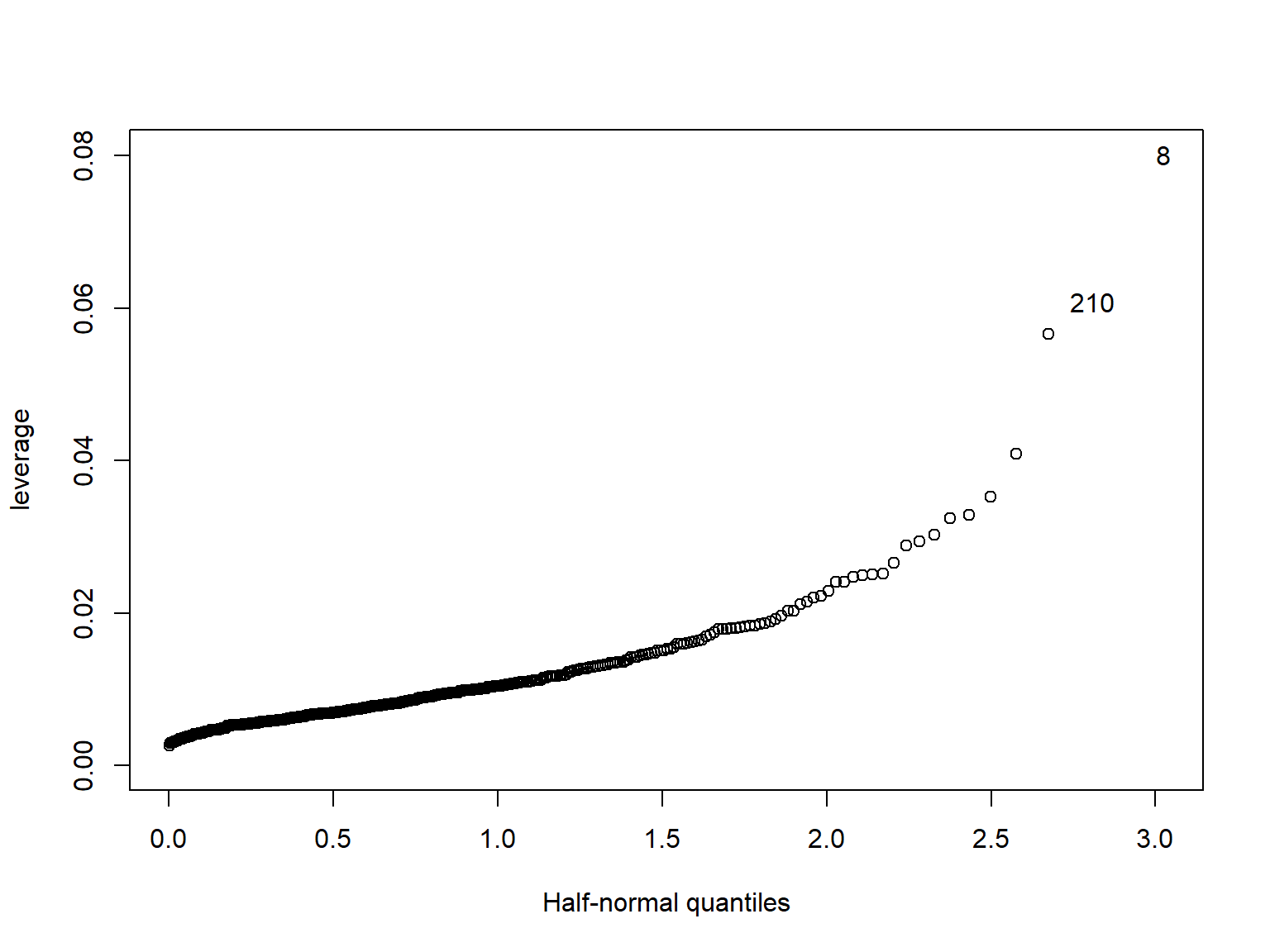Cook’s distance is a measure for influence points. A point with high level of cook’s distance is considers as a point with high influence point. A cut of value for cook’s distance can be calculated as $\frac{4}{(n - p - 1)}$ Where n is sample size and p is number of predictors. We can plot Cook’s distance using the following code: #the cut of value for cook's distance cutoff <- 4/((nrow(d)-length(m2$coefficients)-2))
plot(m2, which = 4, cook.levels = cutoff)